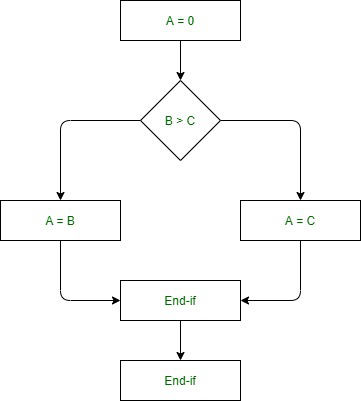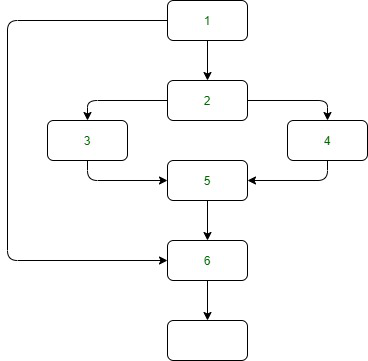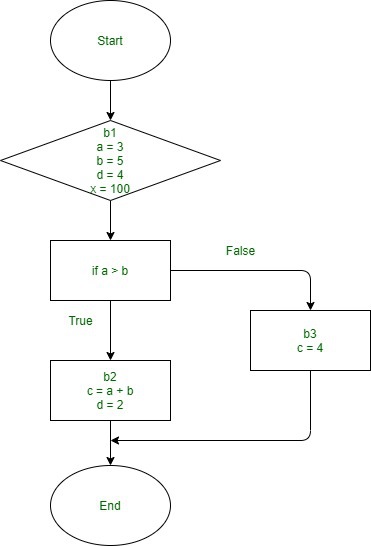GeeksforGeeks App
Open AppBrowser
Continue

# Iterative algorithm for a backward data flow problem

Introduction :
The reason for this article is to inform you approximately an iterative set of rules for backward statistics float problems. Before beginning, you must recognize a few terminologies associated with statistics float analysis.

Data flow analysis :
It is a technique for collecting information about the possible set of values calculated at various points in a computer program.
It is the evaluation of go with the drift of statistics on top of things flow graph, i.e., the evaluation that determines the records concerning the definition and use of statistics in the program. With the assist of this evaluation, optimization may be done. In general, its manner wherein values have computed the use of statistics flow evaluation. The statistics go with the drift property represents records that may be used for optimization.

Control Flow Graph (CFG) :
It is used to decide the elements of the software to which a specific fee assigned to a variable would possibly propagate.
A Control Flow Graph (CFG) is the graphical illustration of managing the flow of computation throughout the execution of packages or applications. Control flow graphs are more often than not utilized in static evaluation in addition to compiler applications, as they are able to appropriately represent the flow internal of an application unit. The manage goes with the drift graph became at the start evolved via way of means of Frances E. Allen.

Example –

```if  A = 10 then
if B > C
A = B
else A = C
endif
endif
print A, B, C ```

Flowchart of the above example –Control Flow Graph of above example –Naive approach ( Kildall’s method ) –
The simplest manner to carry out a data-waft evaluation of packages is to installation data-waft equations for every node of the control-waft graph and clear up them through, again and again, calculating the output from the enter domestically at every node till the complete device stabilizes, i.e., it reaches a fixpoint.

An iterative algorithm –
An iterative set of rules is the maximum not unusual place manner to resolve the facts go with the drift evaluation equations. In this set of rules, we especially have states first one is in-nation and the opposite one is out-nation. The set of rules begins to start with an approximation of the in-nation of every block and is then computed via way of means of making use of the switch capabilities at the in-states. The in-states are up to date via way of means of making use of the be part of operations. The latter steps are repeated till we attain the fixpoint: the scenario wherein the in-states do now no longer change.

The efficiency of the above algorithm –
The efficiency of this algorithm for solving the data-flow equations is influenced by the order in which local nodes are visited and also relies upon whether or not the data-flow equations are used for forwarding or backward data-flow evaluation over the CFG  (manage flow graph).

A few generation orders for fixing data-float equations are mentioned below –

• Random order –
In this new release order isn’t always conscious whether or not the data-waft equations resolve an ahead or backward data-waft problem. And hence, the overall performance is pretty negative in comparison to specialized new release orders.
• Postorder
This new release order for backward data-go with the drift problems. A node is visited in any case its successor nodes were visited, and carried out with the depth-first strategy.
• Reverse postorder
This generation order is for forwarding data-go with the drift problems. The node is visited earlier than any of its successor nodes has been visited, besides whilst the successor is reached via way of means of a returned edge.

Backward analysis –
It is a method to investigate randomized algorithms with the aid of using imagining as though it became running backward in time, from output to input.

Example –
In-state of a block –
The set of variables that contain at the start of it, and It initially contains all variables contained in the block before the transfer function is carried out and the real contained values are computed.

Out-state of a block –
The set of variables that contain at the end of the block and is computed by the union of the block’s successors’ in-states.

```Initial code -
b1: a = 3;
b = 5;
d = 4;
x = 100;
if a > b then
b2:    c = a + b;
d = 2;
b3: endif
c = 4;
return b * d + c;``````Backward data analysis -

// in: {}
b1: a = 3;
b = 5;
d = 4;
x = 100; //x is never being used later thus not in the out set {a,b,d}
if a > b then
// out:     //union of all (in) successors of b1 => b2: , and b3:

//  in: {a,b}
b2: c = a + b;
d = 2;
//  out: {b,d}

//  in: {b,d}
b3: endif
c = 4;
return b * d + c;
//  out:{}```

From the above example, we can observe three points that are –

• Firstly, the in-state of b3 only contains b and d, but instead, c has been written.
• Secondly, the out-nation of b1 is the union of the in-states of b2 and b3.
• The last point is that The definition of c in b2 can be removed since c is not contained immediately after the statement.

The progress table –

Work list –
It is a list of blocks that still need to be processed.

```Note -
The b1 changed into entered withinside the listing earlier than b2, which compelled processing b1
twice (b1 changed into re-entered as
the predecessor of b2).Inserting b2 earlier than b1 could